Search by Topic

Resources tagged with Curious similar to Where to Land:

Filter by: Content type:
Age range:
Challenge level:

There are 68 results

Broad Topics > Habits of Mind > CuriousWhere to Land

Age 14 to 16 Challenge Level:

Chris is enjoying a swim but needs to get back for lunch. If she can swim at 3 m/s and run at 7m/sec, how far along the bank should she land in order to get back as quickly as possible?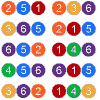Mathsland National Lottery

Age 14 to 16 Challenge Level:

Can you work out the probability of winning the Mathsland National Lottery? Try our simulator to test out your ideas.Age 14 to 16 Challenge Level:

In this follow-up to the problem Odds and Evens, we invite you to analyse a probability situation in order to find the general solution for a fair game.Data Matching

Age 14 to 18 Challenge Level:

Use your skill and judgement to match the sets of random data.Largest Product

Age 11 to 14 Challenge Level:

Which set of numbers that add to 10 have the largest product?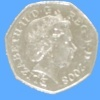Last One Standing

Age 14 to 16 Challenge Level:

Imagine a room full of people who keep flipping coins until they get a tail. Will anyone get six heads in a row?Hexy-metry

Age 14 to 16 Challenge Level:

A hexagon, with sides alternately a and b units in length, is inscribed in a circle. How big is the radius of the circle?Arithmagons

Age 14 to 16 Challenge Level:

Can you find the values at the vertices when you know the values on the edges?Vector Journeys

Age 14 to 18 Challenge Level:

Charlie likes to go for walks around a square park, while Alison likes to cut across diagonally. Can you find relationships between the vectors they walk along?Marbles in a Box

Age 11 to 16 Challenge Level:

How many winning lines can you make in a three-dimensional version of noughts and crosses?Triangle Midpoints

Age 14 to 16 Challenge Level:

You are only given the three midpoints of the sides of a triangle. How can you construct the original triangle?Curvy Areas

Age 14 to 16 Challenge Level:

Have a go at creating these images based on circles. What do you notice about the areas of the different sections?Opposite Vertices

Age 11 to 14 Challenge Level:

Can you recreate squares and rhombuses if you are only given a side or a diagonal?Semi-regular Tessellations

Age 11 to 16 Challenge Level:

Semi-regular tessellations combine two or more different regular polygons to fill the plane. Can you find all the semi-regular tessellations?Age 14 to 16 Challenge Level:

Explore when it is possible to construct a circle which just touches all four sides of a quadrilateral.Who's the Best?

Age 11 to 14 Challenge Level:

Which countries have the most naturally athletic populations?Blue and White

Age 11 to 14 Challenge Level:

Identical squares of side one unit contain some circles shaded blue. In which of the four examples is the shaded area greatest?Think of Two Numbers

Age 11 to 14 Challenge Level:

Think of two whole numbers under 10, and follow the steps. I can work out both your numbers very quickly. How?Same Number!

Age 14 to 16 Challenge Level:

If everyone in your class picked a number from 1 to 225, do you think any two people would pick the same number?Take Three from Five

Age 14 to 16 Challenge Level:

Caroline and James pick sets of five numbers. Charlie chooses three of them that add together to make a multiple of three. Can they stop him?Which Spinners?

Age 14 to 18 Challenge Level:

Can you work out which spinners were used to generate the frequency charts?Pick's Theorem

Age 14 to 16 Challenge Level:

Polygons drawn on square dotty paper have dots on their perimeter (p) and often internal (i) ones as well. Find a relationship between p, i and the area of the polygons.A Little Light Thinking

Age 14 to 16 Challenge Level:

Here is a machine with four coloured lights. Can you make two lights switch on at once? Three lights? All four lights?Cuboid Challenge

Age 11 to 16 Challenge Level:

What's the largest volume of box you can make from a square of paper?Sending a Parcel

Age 11 to 14 Challenge Level:

What is the greatest volume you can get for a rectangular (cuboid) parcel if the maximum combined length and girth are 2 metres?Charlie's Delightful Machine

Age 11 to 16 Challenge Level:

Here is a machine with four coloured lights. Can you develop a strategy to work out the rules controlling each light?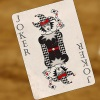Statement Snap

Age 7 to 14 Challenge Level:

You'll need to know your number properties to win a game of Statement Snap...Satisfying Statements

Age 11 to 14 Challenge Level:

Can you find any two-digit numbers that satisfy all of these statements?Cola Can

Age 11 to 14 Challenge Level:

An aluminium can contains 330 ml of cola. If the can's diameter is 6 cm what is the can's height?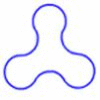Arclets

Age 14 to 16 Challenge Level:

Each of the following shapes is made from arcs of a circle of radius r. What is the perimeter of a shape with 3, 4, 5 and n "nodes".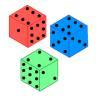Non-transitive Dice

Age 11 to 14 Challenge Level:

Alison and Charlie are playing a game. Charlie wants to go first so Alison lets him. Was that such a good idea?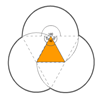Triangles and Petals

Age 14 to 16 Challenge Level:

An equilateral triangle rotates around regular polygons and produces an outline like a flower. What are the perimeters of the different flowers?Cosy Corner

Age 11 to 14 Challenge Level:

Six balls of various colours are randomly shaken into a trianglular arrangement. What is the probability of having at least one red in the corner?Two's Company

Age 11 to 14 Challenge Level:

7 balls are shaken in a container. You win if the two blue balls touch. What is the probability of winning?Estimating Time

Age 11 to 14 Challenge Level:

How well can you estimate 10 seconds? Investigate with our timing tool.Searching for Mean(ing)

Age 11 to 14 Challenge Level:

Imagine you have a large supply of 3kg and 8kg weights. How many of each weight would you need for the average (mean) of the weights to be 6kg? What other averages could you have?Can They Be Equal?

Age 11 to 14 Challenge Level:

Can you find rectangles where the value of the area is the same as the value of the perimeter?Unequal Averages

Age 11 to 14 Challenge Level:

Play around with sets of five numbers and see what you can discover about different types of average...Litov's Mean Value Theorem

Age 11 to 14 Challenge Level:

Start with two numbers and generate a sequence where the next number is the mean of the last two numbers...Stars

Age 11 to 14 Challenge Level:

Can you find a relationship between the number of dots on the circle and the number of steps that will ensure that all points are hit?On the Edge

Age 11 to 14 Challenge Level:

If you move the tiles around, can you make squares with different coloured edges?Shifting Times Tables

Age 11 to 14 Challenge Level:

Can you find a way to identify times tables after they have been shifted up or down?What Numbers Can We Make?

Age 11 to 14 Challenge Level:

Imagine we have four bags containing a large number of 1s, 4s, 7s and 10s. What numbers can we make?A Chance to Win?

Age 11 to 14 Challenge Level:

Imagine you were given the chance to win some money... and imagine you had nothing to lose...Number Pyramids

Age 11 to 14 Challenge Level:

Try entering different sets of numbers in the number pyramids. How does the total at the top change?More Number Pyramids

Age 11 to 14 Challenge Level:

When number pyramids have a sequence on the bottom layer, some interesting patterns emerge...Special Numbers

Age 11 to 14 Challenge Level:

My two digit number is special because adding the sum of its digits to the product of its digits gives me my original number. What could my number be?Reversals

Age 11 to 14 Challenge Level:

Where should you start, if you want to finish back where you started?Pair Products

Age 14 to 16 Challenge Level:

Choose four consecutive whole numbers. Multiply the first and last numbers together. Multiply the middle pair together. What do you notice?What's Possible?

Age 14 to 16 Challenge Level:

Many numbers can be expressed as the difference of two perfect squares. What do you notice about the numbers you CANNOT make?